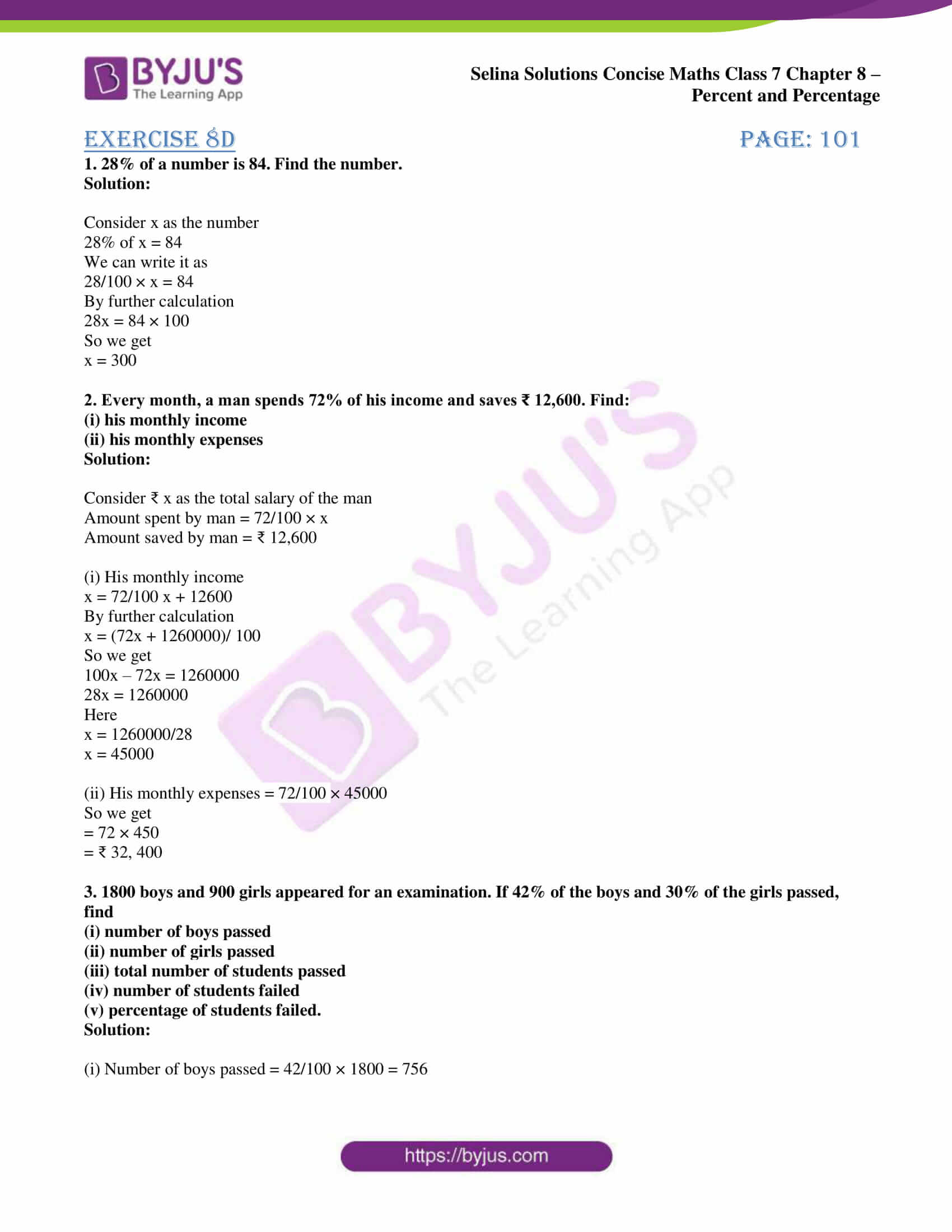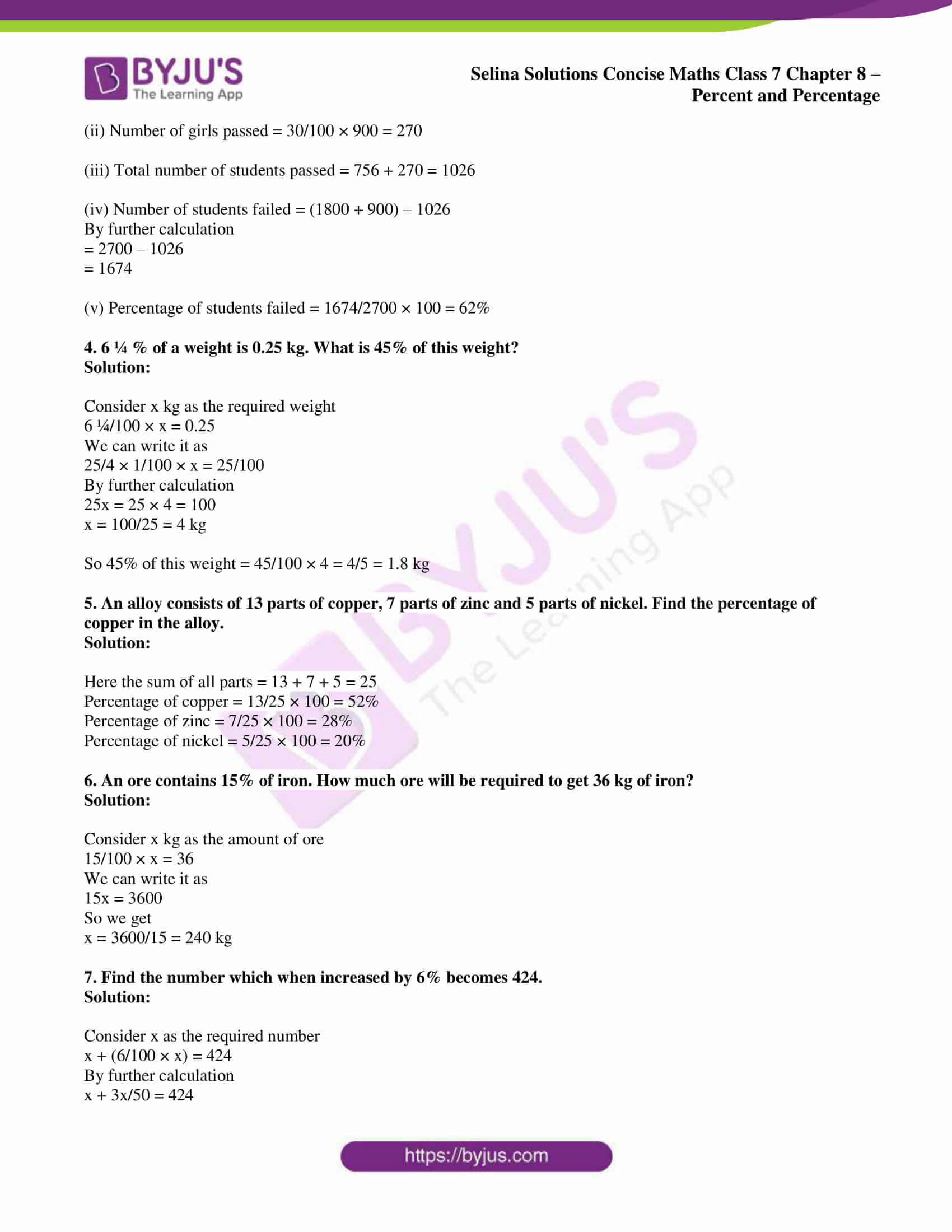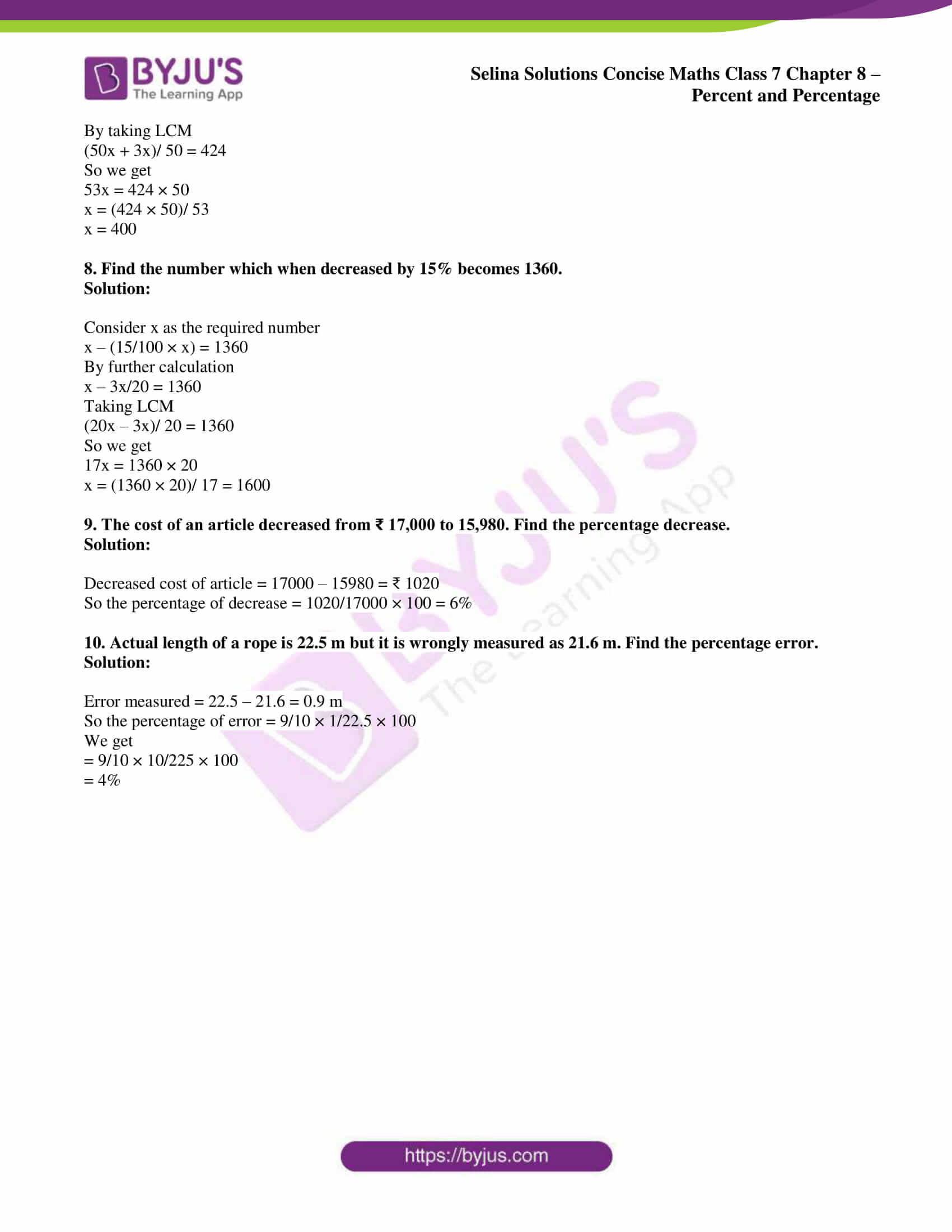# Selina Solutions Concise Maths Class 7 Chapter 8: Percent and Percentage Exercise 8D

Selina Solutions Concise Maths Class 7 Chapter 8 Percent and Percentage Exercise 8D revolves around determining the percentage, which makes the exercise more interesting. Mathematics is a subject which involves numbers where one can play with them to arrive at a specific solution. Solving the exercise wise problems improves time management skills among students, which is important from the exam perspective. Students can download Selina Solutions Concise Maths Class 7 Chapter 8 Percent and Percentage Exercise 8D PDF, from the links which are given here.

## Selina Solutions Concise Maths Class 7 Chapter 8: Percent and Percentage Exercise 8D Download PDF### Access Selina Solutions Concise Maths Class 7 Chapter 8: Percent and Percentage Exercise 8D

#### Exercise 8D page: 101

1. 28% of a number is 84. Find the number.

Solution:

Consider x as the number

28% of x = 84

We can write it as

28/100 × x = 84

By further calculation

28x = 84 × 100

So we get

x = 300

2. Every month, a man spends 72% of his income and saves ₹ 12,600. Find:

(i) his monthly income

(ii) his monthly expenses

Solution:

Consider ₹ x as the total salary of the man

Amount spent by man = 72/100 × x

Amount saved by man = ₹ 12,600

(i) His monthly income

x = 72/100 x + 12600

By further calculation

x = (72x + 1260000)/ 100

So we get

100x – 72x = 1260000

28x = 1260000

Here

x = 1260000/28

x = 45000

(ii) His monthly expenses = 72/100 × 45000

So we get

= 72 × 450

= ₹ 32, 400

3. 1800 boys and 900 girls appeared for an examination. If 42% of the boys and 30% of the girls passed, find

(i) number of boys passed

(ii) number of girls passed

(iii) total number of students passed

(iv) number of students failed

(v) percentage of students failed.

Solution:

(i) Number of boys passed = 42/100 × 1800 = 756

(ii) Number of girls passed = 30/100 × 900 = 270

(iii) Total number of students passed = 756 + 270 = 1026

(iv) Number of students failed = (1800 + 900) – 1026

By further calculation

= 2700 – 1026

= 1674

(v) Percentage of students failed = 1674/2700 × 100 = 62%

4. 6 ¼ % of a weight is 0.25 kg. What is 45% of this weight?

Solution:

Consider x kg as the required weight

6 ¼/100 × x = 0.25

We can write it as

25/4 × 1/100 × x = 25/100

By further calculation

25x = 25 × 4 = 100

x = 100/25 = 4 kg

So 45% of this weight = 45/100 × 4 = 4/5 = 1.8 kg

5. An alloy consists of 13 parts of copper, 7 parts of zinc and 5 parts of nickel. Find the percentage of copper in the alloy.

Solution:

Here the sum of all parts = 13 + 7 + 5 = 25

Percentage of copper = 13/25 × 100 = 52%

Percentage of zinc = 7/25 × 100 = 28%

Percentage of nickel = 5/25 × 100 = 20%

6. An ore contains 15% of iron. How much ore will be required to get 36 kg of iron?

Solution:

Consider x kg as the amount of ore

15/100 × x = 36

We can write it as

15x = 3600

So we get

x = 3600/15 = 240 kg

7. Find the number which when increased by 6% becomes 424.

Solution:

Consider x as the required number

x + (6/100 × x) = 424

By further calculation

x + 3x/50 = 424

By taking LCM

(50x + 3x)/ 50 = 424

So we get

53x = 424 × 50

x = (424 × 50)/ 53

x = 400

8. Find the number which when decreased by 15% becomes 1360.

Solution:

Consider x as the required number

x – (15/100 × x) = 1360

By further calculation

x – 3x/20 = 1360

Taking LCM

(20x – 3x)/ 20 = 1360

So we get

17x = 1360 × 20

x = (1360 × 20)/ 17 = 1600

9. The cost of an article decreased from ₹ 17,000 to 15,980. Find the percentage decrease.

Solution:

Decreased cost of article = 17000 – 15980 = ₹ 1020

So the percentage of decrease = 1020/17000 × 100 = 6%

10. Actual length of a rope is 22.5 m but it is wrongly measured as 21.6 m. Find the percentage error.

Solution:

Error measured = 22.5 – 21.6 = 0.9 m

So the percentage of error = 9/10 × 1/22.5 × 100

We get

= 9/10 × 10/225 × 100

= 4%